Question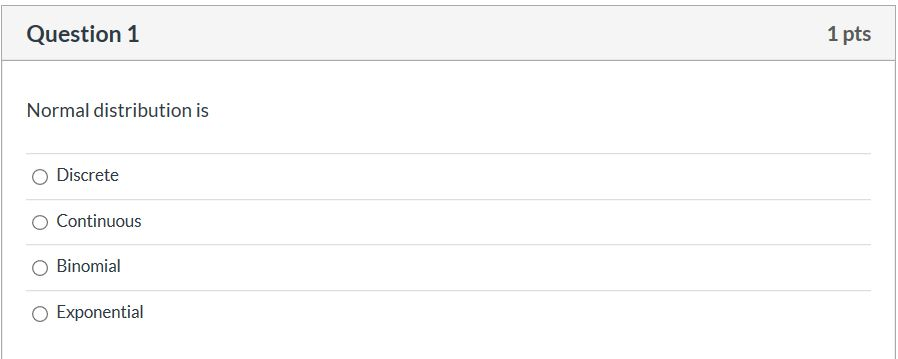The probability density function of the normal distribution is: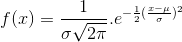Support of the normal distribution is: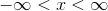So the normal distribution is:

Continuous.

#### Earn Coins

Coins can be redeemed for fabulous gifts.

Similar Homework Help Questions
• ### The uniform, normal, and exponential distributions a. are all continuous probability distributions. b. are all discrete...

The uniform, normal, and exponential distributions a. are all continuous probability distributions. b. are all discrete probability distributions. c. are all the same distributions. d. can be either continuous or discrete, depending on the data.

• ### 62. Suppose X follows a Binomial distribution. Is X continuous or discrete? Explain in your own...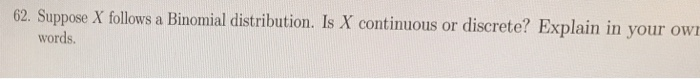62. Suppose X follows a Binomial distribution. Is X continuous or discrete? Explain in your own words.

• ### If requirements hold true, the distribution of the sample means is, Exponential, Uniform, Normal, OR Discrete?

If requirements hold true, the distribution of the sample means is, Exponential, Uniform, Normal, OR Discrete?

• ### What kind of distributions are the binomial and Poisson probability distributions? A. Discrete B. Continuous  ...

What kind of distributions are the binomial and Poisson probability distributions? A. Discrete B. Continuous   C. Both discrete and continuous D. Neither discrete or continuous

• ### (3.4) This question is about a continuous probability dis- tribution known as the exponential distribution Let...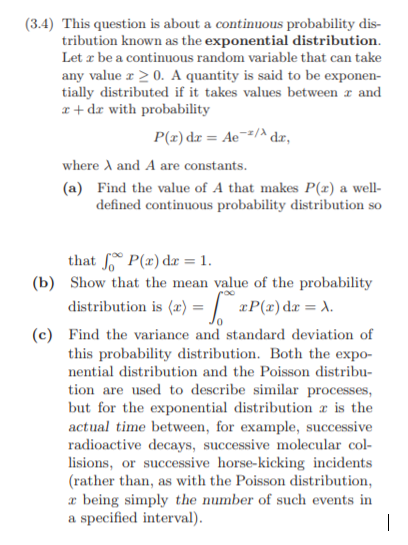(3.4) This question is about a continuous probability dis- tribution known as the exponential distribution Let x be a continuous random variable that can take any value x 20. A quantity is said to be exponen- tially distributed if it takes values between r and r + dr with probability where A and A are constants. (a) Find the value of A that makes P() a well- defined continuous probability distribution so that Jo o P(x) dx = 1 (b)...

• ### A binomial distribution has p=o.22 and n=98. Use the normal approximation to the binomial distribution to...

A binomial distribution has p=o.22 and n=98. Use the normal approximation to the binomial distribution to answer parts a through d. a. what are the mean and standard deviation for this distribution? b. what us the probability of exactly 16 successes? c. what is the probability of 14 to 25 successes? d. what is the probability of 12 to 20 successes?

• ### Question 3 20 pts Fill the blanks With: Erlang, Lamda/unit time, Exponential, Poisson, Continuous: Poisson Bernoulli...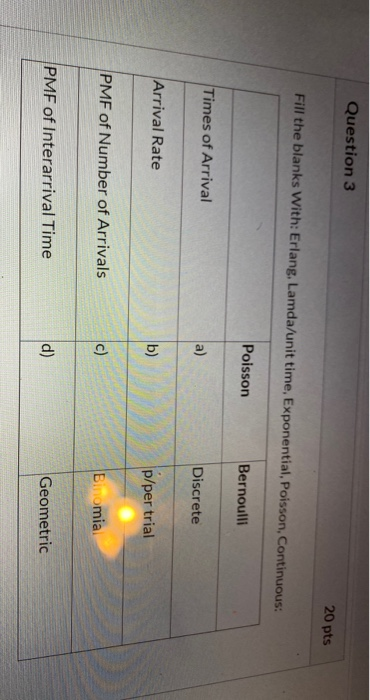Question 3 20 pts Fill the blanks With: Erlang, Lamda/unit time, Exponential, Poisson, Continuous: Poisson Bernoulli Times of Arrival a) Discrete Arrival Rate b) p/per trial PMF of Number of Arrivals c) Binomia PMF of Interarrival Time d) Geometric

• ### (19) For the following discrete randon variables, find m1, m2, and σ (a) Bernoulli (b) Binomial (c) Poisson (d) Geometric (20) For the following continuous random variables, find m1, m2, and σ2 (a) U...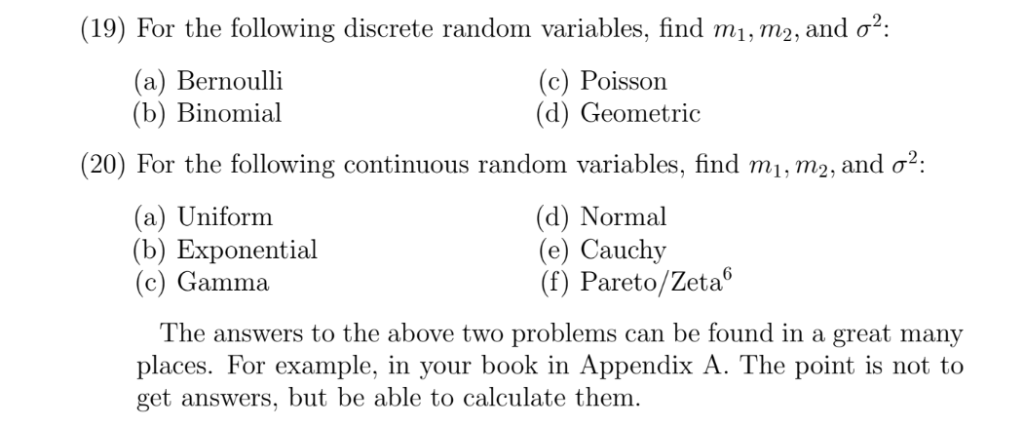(19) For the following discrete randon variables, find m1, m2, and σ (a) Bernoulli (b) Binomial (c) Poisson (d) Geometric (20) For the following continuous random variables, find m1, m2, and σ2 (a) Uniform (b) Exponential (c) Gamma (d) Normal (e) Cauchy. .G (f) Pareto/Zeta" The answers to the above two problems can be found in a great man places. For example, in your book i get answers, but be able to calculate them n Appendix A. The point is...

• ### fundamentals of bios D Question 4 1 pts When deciding if the distribution of a binomial...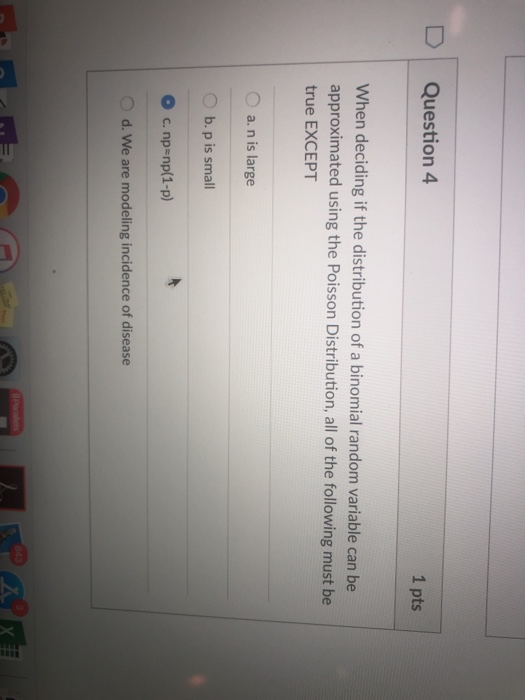fundamentals of bios D Question 4 1 pts When deciding if the distribution of a binomial random variable can be approximated using the Poisson Distribution, all of the following must be true EXCEPT O a. n is large O b. p is small О с. npenp(1-p) d. We are modeling incidence of disease

• ### Question 3 1 pts Determine whether the distribution is a discrete probability distribution. If not, state...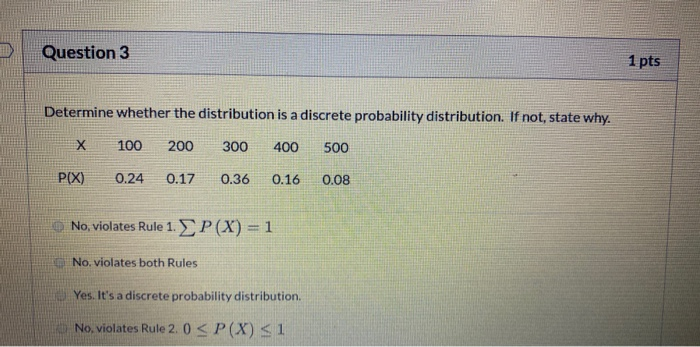Question 3 1 pts Determine whether the distribution is a discrete probability distribution. If not, state why. X 100 200 300 400 500 P(X) 0.24 0.17 0.36 0.16 0.08 No, violates Rule 1. P(X) = 1 No violates both Rules Yes. It's a discrete probability distribution No, violates Rule 2.0 < P(X) 51